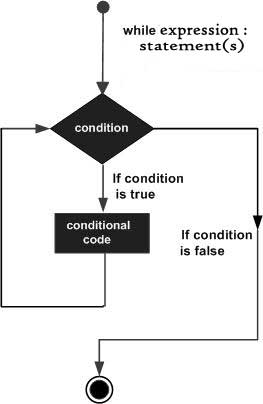# Python while Loop Statements

A while loop statement in Python programming language repeatedly executes a target statement as long as a given condition is true.

### Syntax

The syntax of a while loop in Python programming language is −

```while expression:
statement(s)
```

Here, statement(s) may be a single statement or a block of statements. The condition may be any expression, and true is any non-zero value. The loop iterates while the condition is true.

When the condition becomes false, program control passes to the line immediately following the loop.

In Python, all the statements indented by the same number of character spaces after a programming construct are considered to be part of a single block of code. Python uses indentation as its method of grouping statements.

### Flow DiagramHere, key point of the while loop is that the loop might not ever run. When the condition is tested and the result is false, the loop body will be skipped and the first statement after the while loop will be executed.

### Example

Live Demo
```#!/usr/bin/python

count = 0
while (count < 9):
print 'The count is:', count
count = count + 1

print "Good bye!"
```

When the above code is executed, it produces the following result −

```The count is: 0
The count is: 1
The count is: 2
The count is: 3
The count is: 4
The count is: 5
The count is: 6
The count is: 7
The count is: 8
Good bye!
```

The block here, consisting of the print and increment statements, is executed repeatedly until count is no longer less than 9. With each iteration, the current value of the index count is displayed and then increased by 1.

## The Infinite Loop

A loop becomes infinite loop if a condition never becomes FALSE. You must use caution when using while loops because of the possibility that this condition never resolves to a FALSE value. This results in a loop that never ends. Such a loop is called an infinite loop.

An infinite loop might be useful in client/server programming where the server needs to run continuously so that client programs can communicate with it as and when required.

```#!/usr/bin/python

var = 1
while var == 1 :  # This constructs an infinite loop
num = raw_input("Enter a number  :")
print "You entered: ", num

print "Good bye!"
```

When the above code is executed, it produces the following result −

```Enter a number  :20
You entered:  20
Enter a number  :29
You entered:  29
Enter a number  :3
You entered:  3
Enter a number between :Traceback (most recent call last):
File "test.py", line 5, in <module>
num = raw_input("Enter a number :")
KeyboardInterrupt
```

Above example goes in an infinite loop and you need to use CTRL+C to exit the program.

## Using else Statement with While Loop

Python supports to have an else statement associated with a loop statement.

• If the else statement is used with a while loop, the else statement is executed when the condition becomes false.

The following example illustrates the combination of an else statement with a while statement that prints a number as long as it is less than 5, otherwise else statement gets executed.

Live Demo
```#!/usr/bin/python

count = 0
while count < 5:
print count, " is  less than 5"
count = count + 1
else:
print count, " is not less than 5"
```

When the above code is executed, it produces the following result −

```0 is less than 5
1 is less than 5
2 is less than 5
3 is less than 5
4 is less than 5
5 is not less than 5
```

## Single Statement Suites

Similar to the if statement syntax, if your while clause consists only of a single statement, it may be placed on the same line as the while header.

Here is the syntax and example of a one-line while clause −

```#!/usr/bin/python

flag = 1
while (flag): print 'Given flag is really true!'
print "Good bye!"
```

It is better not try above example because it goes into infinite loop and you need to press CTRL+C keys to exit.

python_loops.htm